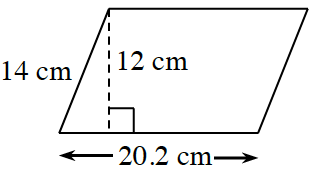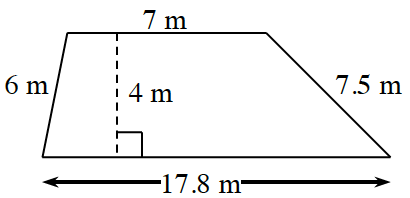### Home > CC1 > Chapter 7 > Lesson 7.3.1 > Problem7-90

7-90.

Find the area and perimeter of each figure below.

1.The formula to find the area of a parallelogram is the same as the formula to find the area of a rectangle:
(base)(height).

$\text{Perimeter} = 2(20.2 \text{ cm}) + 2(14 \text{ cm}) \\= 68.4 \text{ cm}$

$\text{Area} = (20.2 \text{ cm})(12 \text{ cm})\\ = 242.4 \text{ cm}^2$

1.Use the formula below to find the area of a trapezoid:

$\text{Area}=(\text{height}) \! \left ( \frac{\text{base}_{1}+\text{base}{2}}{2} \right )$